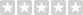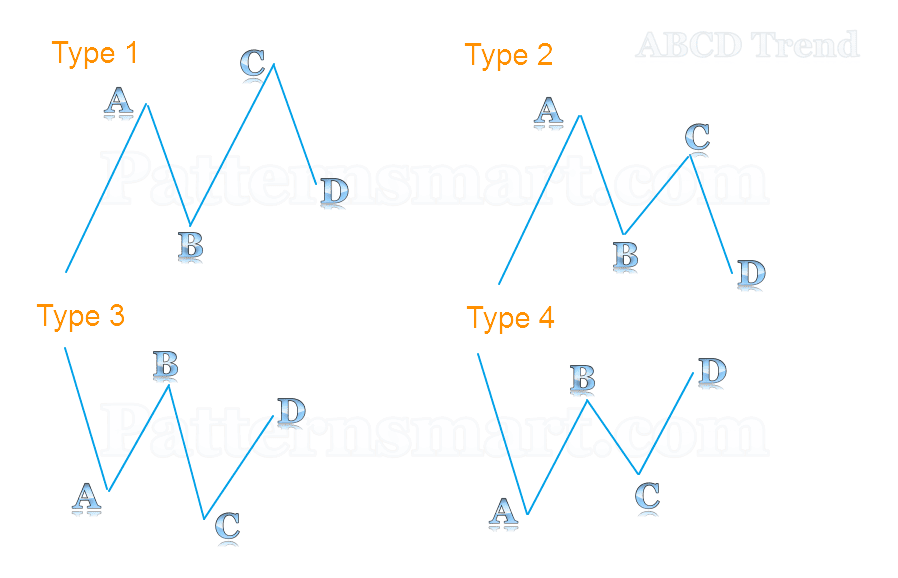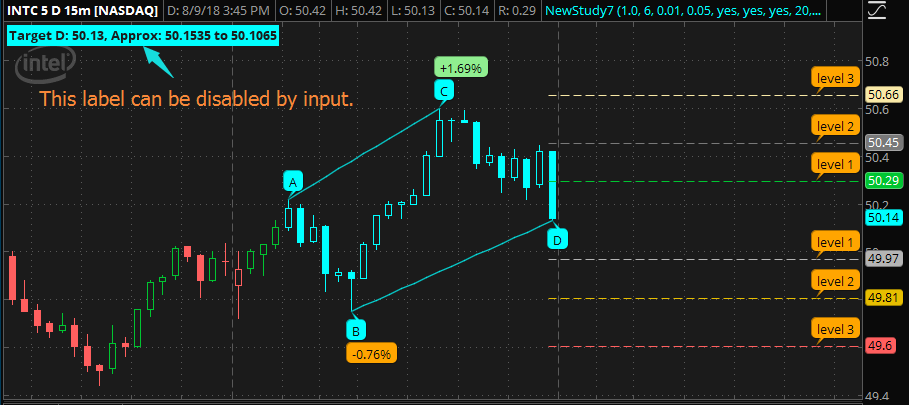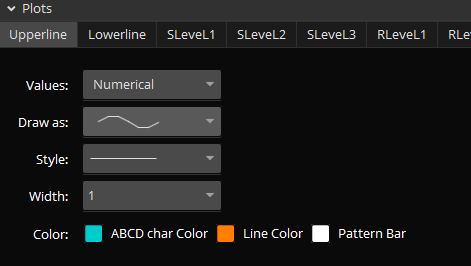Popular tags: Support, Resistance, Trend, Range, Fibonacci, Wave, Divergence, Multi time frames(MTF), Swing, Volume, Overbought, Oversold

Featured

# ABCD trend chart pattern indicator and scan for Thinkorswim TOS

Availability: Special order
Price: \$450.00USD
Qty:     - OR -(0/5)  0 reviews  |  Write a review

## Please make sure you understand the mechanism before making purchase.

What's the ABCD trend chart pattern?

Our definition is when A, B and C are swing points and D reaches a given ratio of AB:CD.

The ratio of AB:CD is determined by comparing the price difference between C to D and A to B.

Here is the formula to calculate it: |Price of C - Price of D| / |Price of A - Price of B|.

I categorized four types of ABCD trend chart pattern.

• Type1 is when point A and C are swing highs and point B is swing low, point C is higher than point A, point D can be either higher or lower than point B.
• Type2 is when point A and C are swing highs and point B is swing low, point C is lower than point A, point D can be either higher or lower than point B.
• Type3 is when point A and C are swing lows and point B is swing high, point C is lower than point A, point D can be either higher or lower than point B.
• Type4 is when point A and C are swing lows and point B is swing high, point C is higher than point A, point D can be either higher or lower than point B.In order to be more efficient, I created two indicators for the ABCD trend chart pattern, one for downtrend which is used to detect pattern type 1 and 2, one for uptrend which is used to detect pattern type 3 and 4.

How it works?

The point A,B and C are swing points, once these three points are formed and if current bar was qualified to be point D the indicator will send a sound alert and highlight the pattern on chart. It will also plot six support and resistance levels, the default levels are based on golden ratio, you can change the ratios from input.

You will be able to control the shape of the pattern in order to detect your desired ones only.

If you want AB=CD, then just set the ABCD ratio input to 1.

You can also use different value for ABCD ratio input, such as 1.2 which means  |Price of C - Price of D| / |Price of A - Price of B|≈1.2, or using 0.618 for ABCD ratio which means  |Price of C - Price of D| / |Price of A - Price of B| ≈ 0.618.

In the equation above, I used the symbol of approximately equal (≈) because the exact ratio value only exists in theory. We can hardly find any match without a threshold. The ratio value will be calculated with a very small threshold in order to get more results.Please note that this indicator is not intended for trend prediction,  it will not info you the direction of the price.## Both indicators have the following parameters and features.

ABCD ratio, this parameter is used to adjust the ratio of AB:CD.

Strength, this parameter is used to define the minimum number of bars to the left and right side of the swing point. For more details of this input please check: https://goo.gl/sWBH7k

AB percent, this parameter is used to check the price difference between A and B in percentage. For example, if you set it to 5, then point B must fall/raise at least 5% from point A.

No repaint if the pattern was drawn after the poiont D closed.

Sound alert when pattern is detected with switch.

Choose different color to highlight the price bars which formed the pattern.

Choose different color for the line that linked AC and BD, and the A,B,C,D char color.## Write a review

Your Review: Note: HTML is not translated!

Enter the code in the box below:Latest
Welcome to Patternsmart
Converting your indicator from one platform to another.